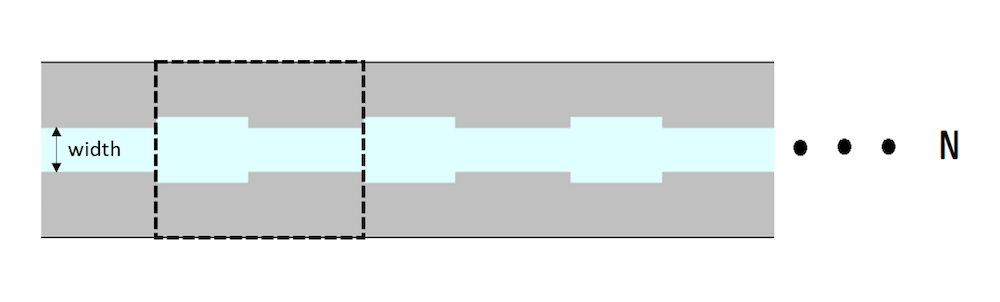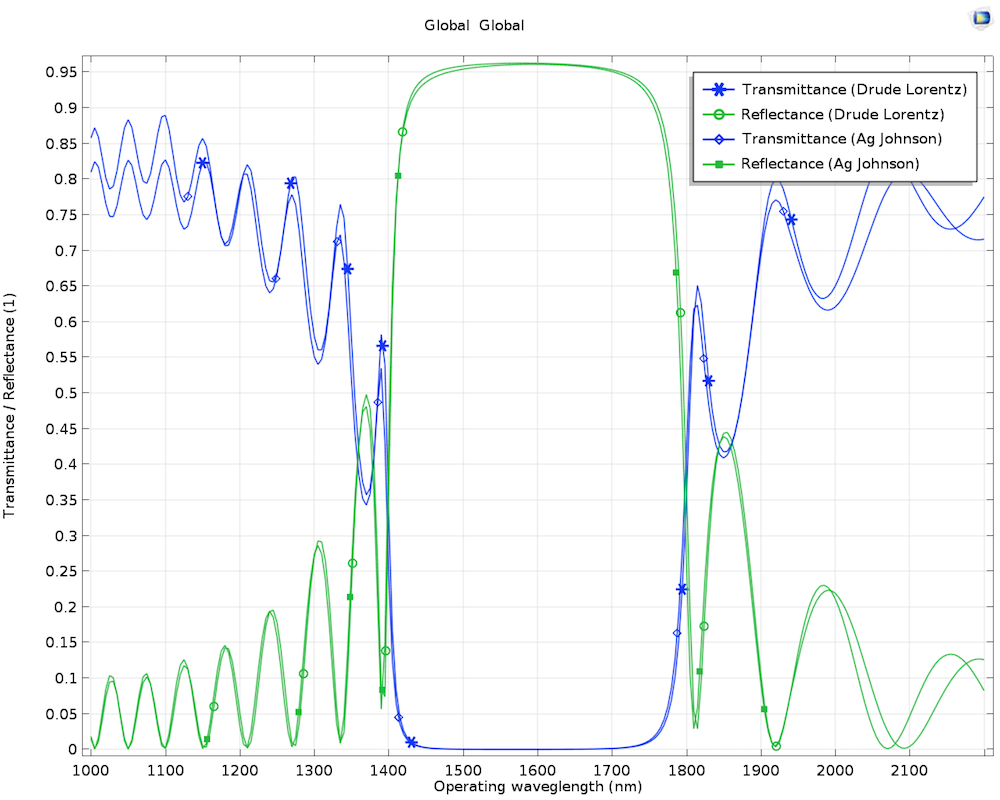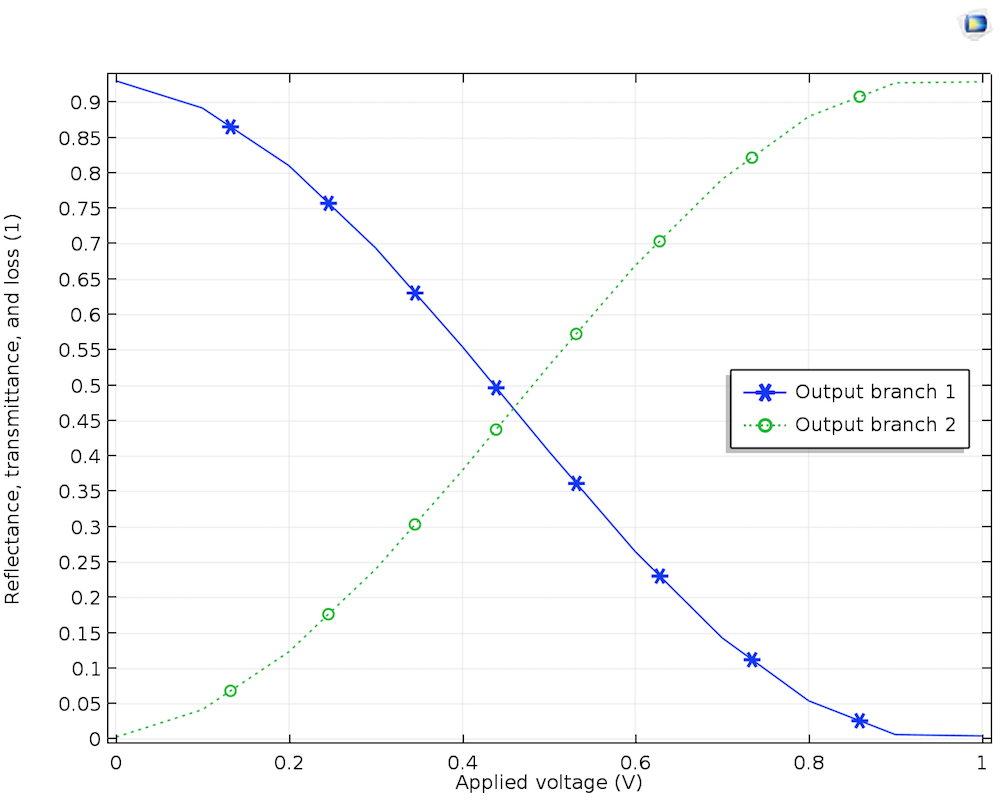如何在 COMSOL 软件中建立线性和非线性光学模型

2018年 4月 6日

1875 年，John Kerr 将载流线圈放置在玻璃板两侧的孔中，从而产生了电场。当一束偏振光穿过平板后，他发现偏振是不同的。这种差异与玻璃折射率的变化有关，折射率与电场的平方成正比——这种现象被称为磁光克尔效应（Kerr effect）今天的博文将带您了解如何对这种效应以及其他线性和非线性现象进行建模。

理解非线性光学材料的磁化率

\textbf{D}= \epsilon_{0} \textbf{E} + \textbf{P} = \epsilon_{0}\textbf{E} + \epsilon_{0} \chi_{0} \textbf{E}

\textbf{P} = \epsilon_0 \begin{bmatrix}
\chi_{11} & \chi_{12} & \chi_{13} \\
\chi_{21} & \chi_{22} & \chi_{23} \\
\chi_{31} & \chi_{32} & \chi_{33}
\end{bmatrix} \textbf{E}

P(\omega) = \epsilon_0 (\chi^
{(1)}(\omega)E(\omega)\chi^{(2)}(\omega)E^2(\omega)\chi^{(3)}(\omega)E^3(\omega)+ . . .)

光学材料的一阶磁化率

\epsilon_r = \epsilon_{\infty}+\frac{\omega_p^2} {\omega_0^2-\omega^2+i\Gamma\omega}

模拟等离子体波导滤波器光学材料的二阶磁化率

SHG 被应用于激光设计和工程领域，在这个领域，很难找到一种材料来发射比入射波长波长更短的光。例如，当红外光源(1064nm)通过磷酸二氢钾(KDP)晶体泵浦时，晶体会发射出绿色(532nm)的激光源。

D = \epsilon_0 \epsilon_r E + D_r

P_{1y}= 2d E_{2y} E_{1y}^*
P_{2y}= d E_{1y}^2

y-基频电场分量，E_{2y}y-二次谐波频率下的电场分量。

光学材料的三阶磁化率

n = n_0 + \gamma I

具有电光效应的材料

n(E) = n + \alpha_1 E + \frac{1}{2} \alpha_2 E^2 + . . .

n(E) = n + \frac{1} {2}d_1 n^3 E + \frac{1}{2}
d_2 n^3 E^2 + . . .

关于泡克耳斯效应（Pockels Effect）

n(E) = n + \frac{1}{2}d_1 n^3 E关于克尔效应

n(E) = n + \frac{1} {2}d_2 n^3 E^2

下一步

1. J. Leuthold, C. Koos, and W. Freude, “Nonlinear silicon photonics”, NaturePhotonics, vol. 4, pp. 535–544, 2010.
2. Z. Han, E. Forsberg, and S. He, “Surface Plasmon Bragg Gratings Formed in Metal-Insulator-Metal Waveguides,” IEEE Photonics Technology Letters, vol. 19, no. 2, pp. 91–93, Jan. 15, 2007.
3. B. E. A. Saleh and M. C. Teich, Fundamentals of Photonics, John Wiley & Sons, Inc.
4. P. B. Johnson and R. W. Christy, “Optical constants of the noble metals,” Phys. Rev. B, vol. 6, no. 12, pp. 4370–4379, Dec. 15, 1972.
5. Y. R. Shen, The Principles of Nonlinear Optics, John Wiley & Sons, Inc.